# Algorithms on Graphs: Directed Graphs and Cycle Detection

By now, we have an understanding of what a graph is and learned some of the methods in traversing them.

While, we’ve already seen directed graphs, it’s time to get a formal understanding of them and uncover exciting properties that come along with their nature.

In this post, we’ll be exploring directed graphs along with learning how to determine if they have cycles within them.

Let’s first start off with learning what a directed graph is.

# Directed Graphs

Directed graphs are graphs where edges go in one direction. Here’s an example.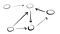Notice how every edge is pointing in one direction to another vertex

As we can see, we use arrows to indicate that an edge is directed. But how does this differ from a non-directed graph? Let’s look at a comparison: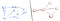Directed (Left) vs. Non-directional (Right)

In the directed graph, the arrows indicates that you can go one way but not back unless you go through a different edge. While in a non-directed graph, we use lines to show that we can go back and forth using the same edge.

Note: You will see explanations around using the terms “non-directed” or “bidirectional”. They mean the same thing. Basically, you can go back and forth between vertices using the same edge. You can’t do that in a directed graph.

A more tangible example to illustrate the difference between directed and non-directed / bidirectional graphs is that directed edges can be thought of as one way streets while non-directed ones can be thought of as two-way roads: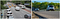(Left) One-way streets vs (Right) Two-way roads

Okay, now that we have a solid understanding of directed graphs, let’s talk about cycles in graphs.

# Cyclic Graphs

Cyclic is a term used to describe a graph with cycles. Think circles. Let’s look at an example of what that graph would look like:

Basically, for a graph to have a cycle, there needs to be at least one path in which one can travel down and return to their starting point. While not every vertex has to have a path that returns to it, there just has to be at least one in the graph in order for the whole graph to be considered cyclic.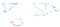More examples of cyclic graphs

Great! Now that we’ve learned about cyclic graphs, let’s learn about graphs without cycles.

# Acyclic Graphs

The term acyclic means “without cycles”.

Acyclic graphs are graphs in which no vertex can come back to itself regardless of the path taken. These graphs are unique to directed graphs because if we recall from earlier, non-directed graphs have edges that act as two way paths. This is fact is so significant that they are even given a name: directed acyclic graphs (DAGs).

Here are a few examples of a DAG: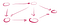Starting at any vertex, there is no path from that vertex that comes back to it

# Cycle Detection

Determining a cycle is pretty straight forward. As we explore a path, if at some point we circle back to a vertex in that path before we finished exploring the entire path, then we have detected a cycle. Let me illustrate what that means:

Suppose we have a graph that looks like this:

We can see that this graph indeed has a cycle (1 → 4 → 5 → 6 → 4). In order to show that this cycle exists, we’ll use DFS to go down a path until we either reach a dead end or come to a vertex that’s in our path.

Assuming that we’re starting at 1, let’s do a DFS and use green to indicate our current path and any vertex that’s colored green means that it’s on that path.

As we hit 3, we’ve reached a dead-end because 3 doesn’t have any edges that point out of it. So, we mark 3 as blue to mean that it has been visited and then retrace back. We’ll then mark 2 as visited because it doesn’t have any other edges going out of it.

Notice how 1 is still green. That’s because we haven’t finished exploring all the paths from 1. Let’s continue down its other neighbor:

Notice at this point, we visit 3 again since it’s a neighbor of 4.

The thing I want to stress is that any vertex that has been marked as visited means that we’ve explored every path from that vertex. Since we didn’t find a cycle, we can assume that when we come across a visited vertex, we can ignore it because we already know that it doesn’t have a cycle. Let’s continue with the process:

As we move further down the path, we see that from 6, we come back to 4. Vertex 4 has not been marked as visited and is currently on our current exploration path. Since we came back to it, we know that we have found a cycle.

Here’s the code in JavaScript:

Since we’re just doing DFS and looking at all the vertices along with their edges, we have a runtime of O(V + E) with a space complexity of O(V + E) as well.

# Summary

• Directed graphs have edges that point from one vertex to another. One can only go one direction on an edge.
• Non-directed / bidirectional graphs have edges where you can go back and forth between vertices.
• Cyclic graphs are graphs with cycles. Basically, there is at least one path in the graph where a vertex can come back to itself.
• Acyclic graphs don’t have cycles.
• Directed acyclic graphs (DAGs) are specific names given to acyclic graphs.
• We can determine if a graph has a cycle by doing DFS and see if we re-explore a vertex that’s on our current exploration path.

## More from Try Khov

Software Engineer